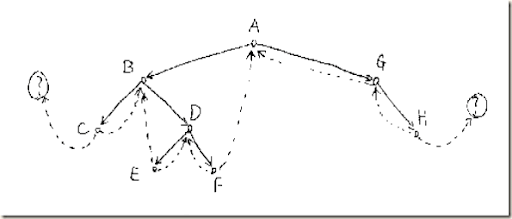## Wednesday, 8 June 2011

### Convert a binary tree to threaded binary trees

“We know the important property of binary tree. Each level has 2^n nodes. If that is the case, what is the number of empty nodes in the leaves. empty nodes mean left or right null near the tree leaves. If its a perfectly balanced tree, you get around 2^<height of the tree> nodes. Which is huge!! and goes for a big waste!!”

See first

Introduction

This is another memory optimization concept where you need to reuse unused space present in the linked list and get easy traversal to parents. And remember the complex problem you would have heard about,

Converting a binary tree to a doubly linked list in-order way without using extra space & reusing the empty left& rights.

This problem is simply nothing but making a binary tree a threaded binary tree with some extra linkages done for getting the doubly linked list property.

There is one more problem for more interested people,

http://www.careercup.com/question?id=3424767

Find the pre-order traversal of a tree without using recursion, stack, extra memory. You can reuse the space already present in the tree and create temporary nodes if required.

There are many applications & interview questions like this which requires prior & complete knowledge of threaded binary trees.

Concept

How threading is done? We know that around 2^<height of the tree> nodes are empty. Which mostly constitutes 50% of the nodes in the tree. There is a mathematical proof for this.

``` Let a null LEFT reference point to the predecessor in in order, and
let a null RIGHT reference point to the successor in in order.```
``